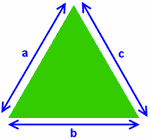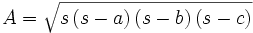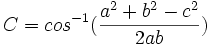# Heron's Formula## Area of a Triangle from Sides

You can calculate the area of a triangle if you know the lengths of all three sides, using a formula that has been known for nearly 2000 years.

It is called "Heron's Formula" after Hero of Alexandria (see below)

Just use this two step process:

Step 1: Calculate "s" (half of the triangles perimeter):

s = a+b+c2

Step 2: Then calculate the Area:### Example: What is the area of a triangle where every side is 5 long?

Step 1: s = 5+5+52 = 7.5
Step 2: A = √(7.5 × 2.5 × 2.5 × 2.5) = √(117.1875) = 10.825...

Try it yourself:

## Angles

In the calculator above I also used the Law of Cosines to calculate the angles (for a complete solution). The formula is:Where "C" is the angle opposite side "c".